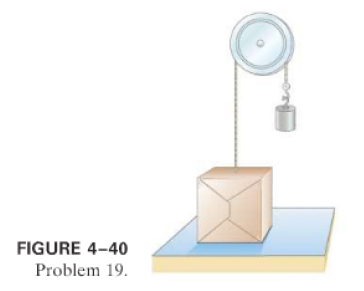×
Get Full Access to Physics: Principles With Applications - 6 Edition - Chapter 4 - Problem 19p
Get Full Access to Physics: Principles With Applications - 6 Edition - Chapter 4 - Problem 19p

×

# (I) A box weighing 77.0 N rests on a table. A rope tied toISBN: 9780130606204 3

## Solution for problem 19P Chapter 4

Physics: Principles with Applications | 6th Edition

• Textbook Solutions
• 2901 Step-by-step solutions solved by professors and subject experts
• Get 24/7 help from StudySoup virtual teaching assistantsPhysics: Principles with Applications | 6th Edition

4 5 1 265 Reviews
14
3
Problem 19P

(I) A box weighingrests on a table. A rope tied to the box runs vertically upward over a pulley and a weight is hung from the other end (Fig. 4-40). Determine the force that the table exerts on the box if the weight hanging on the other side of the pulley weighsa), and.Step-by-Step Solution:

Solution 19P: We have find out the force exerted by the table on the box under the application of weight in various denomination, in a pulley-rope system. Step 1 of 5 Concept: Newton’s second law: The force F acts on mass m produces an acceleration a in the object. Mathematically, F=ma Newton’s third law: To every action, there is an equal and opposite reaction.

Step 2 of 5

Step 3 of 5

##### ISBN: 9780130606204

Unlock Textbook Solution